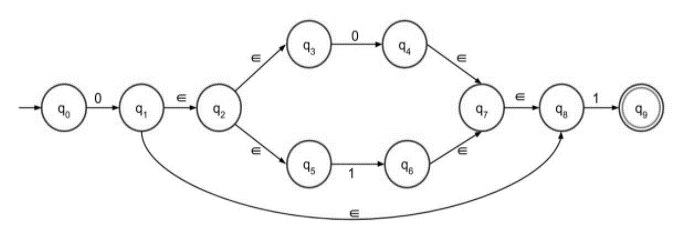# Construct ∈-NFA of Regular Language L = 0(0+1)*1

The ∈ transitions in Non-deterministic finite automata (NFA) are used to move from one state to another without having any symbol from input set Σ

∈-NFA is defined in five tuple representation

{Q, q0, Σ, δ, F}

Where,

• δ − Q × (Σ∪∈)->2Q

• Q − Finite set of states

• Σ − Finite set of the input symbol

• q0 − Initial state

• F − Final state

• δ: Transition function

## NFA without ε transition

NFA also has five states same as DFA, but with different transition function, as shown follows −

$$\delta\colon\:Q\times\:\sum\longrightarrow\:2^{Q}$$

Where,

• Q − Finite set of states

• Σ − Finite set of the input symbol

• q0 − Initial state

• F − Final state

• δ − Transition function

## Example

Consider NFA with ε as follows −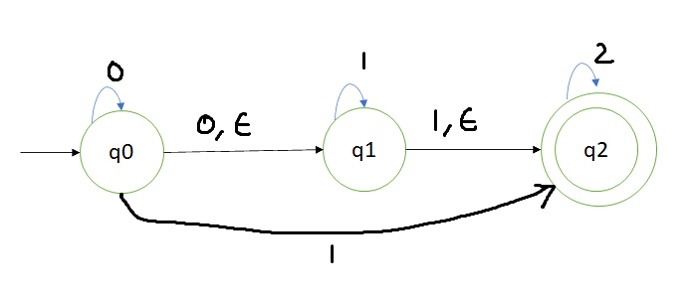Here q0 is a start state with input 0, one can be either in state q0 or in state q1.

If we get at the start symbol 1 then with epsilon transition, we can change state from q0 to q1 and then input 1 can be in state q1.

On the other hand, from state q0 with input 1, we can reach state q2.

Thus, it is not sure that on input 1 whether we will be in state q1 or q2.

Hence it is called non deterministic finite automata and since there are some epsilon moves by which we can simply change the state from 1 to other.

Hence it is called NFA with epsilon.

Let’s consider the given language L = 0(0+1)*1.

## Construct ε-NFA

Steps for construction for ε-NFA are as follows −

Step 1 − NFA with epsilon for 0+ is as follows −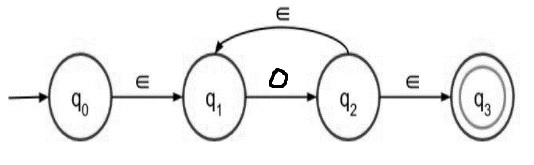Step 2 − NFA with epsilon for 0* is as follows −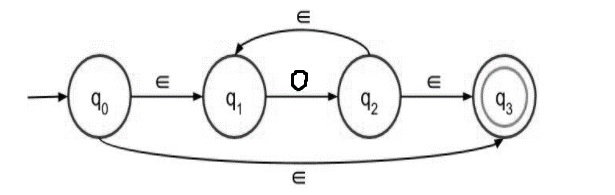Step 3 − NFA with epsilon for (0+1) is as follows −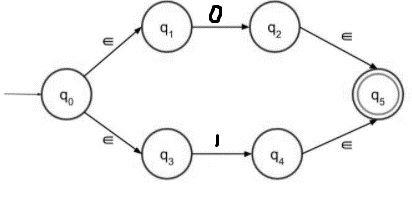The above transition diagram accepts either 0 or 1 as input. Those two paths lead to the final state.

Step 4 − NFA with epsilon for 01 is as follows −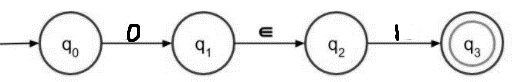For concatenation 0 must be followed by 1.

Step 5 − ε-NFA for L = 0(0+1)*1 is as follows −

L = 0(0+1)*1 is divided into three parts- 0, (0+1)* and 1. The second part, (0+1)*, is constructed above just concatenate with 0 and 1, followed by second rule 0*.

The final NFA with epsilon move is as follows −